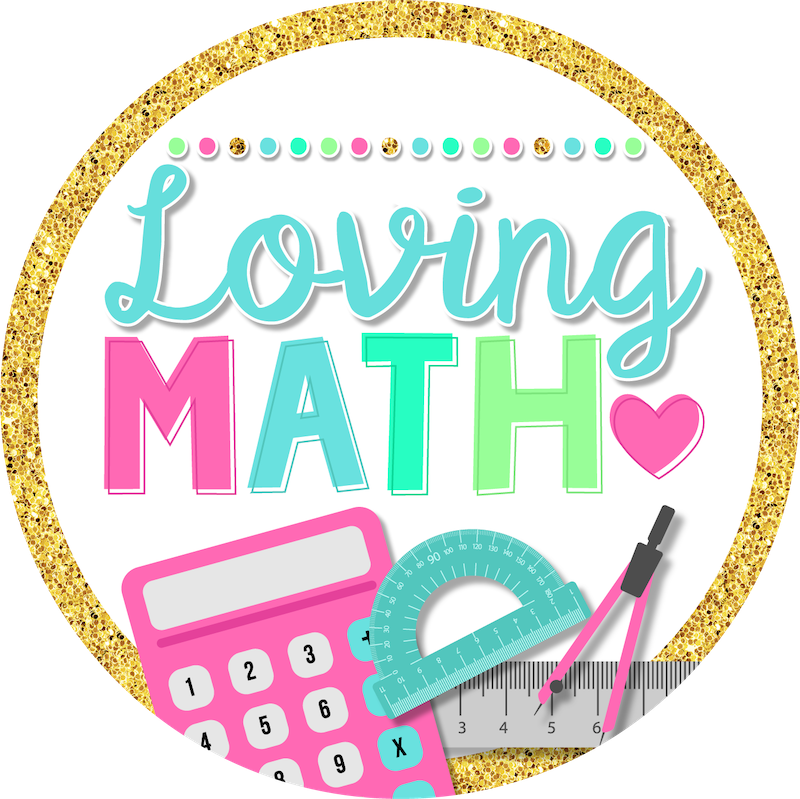# Place Value Games, Crafts, and Activities

Let’s get one thing straight. Place value is a BIG deal!
It is usually the first skill taught in every grade, in every curriculum, at the beginning of every school year. As a result, teachers scramble to find engaging and age appropriate activities for their students.
The common core standards dedicate a huge number of standards to place value, particularly in the early grades.
Why?
Because place value sets the foundation for understanding of numbers in our number system.

#### Look at all the place value standards listed from K to fifth grade:

•Compose and decompose numbers from 11 to 19 into tens and ones. (K)
•Understand a two-digit number as represented by amounts of tens and ones. (1)
•Understand 10 can be thought of as a bundle of 10 ones—called a “ten.” (1)
•Understand a three-digit number as represented by amounts of hundreds, tens, and ones. (2)
•Read and write numbers to 1,000. (2)
•Compare two three-digit numbers. (2)
•Round whole numbers to the nearest 10 or 100. (3)
•Recognize that in a multi digit whole number, a digit in one place represents 10 times what it represents in the place to its right. (4)
•Read and write multi digit whole numbers. (4)
•Compare two multi digit numbers. (4)
•Round multi digit whole numbers to any place. (4)
•Recognize that in a multi digit number, a digit in one place represents 10 times as much as it represents in the place to its right and 1/10 of what it represents in the place to its left. (5)
•Explain patterns in the number of zeros of the product when multiplying a number by powers of 10. (5)
•Explain patterns in the placement of the decimal point when a decimal is multiplied or divided by a power of 10. (5)
•Use whole-number exponents to denote powers of 10. (5)
•Read, write, and compare decimals to thousandths. (5)
•Compare two decimals to thousandths. (5)
•Round decimals to any place. (5)
But teaching place value doesn’t have to be boring. Children should be exposed to plenty of hands on activities, manipulatives, and games to fully understand this very important concept.

### DEVELOPING CONCEPTUAL UNDERSTANDING

Base-10 blocks can be used to help students understand the concepts behind place value. Base-10 blocks also can be used to explain decimals. Other place-value manipulatives are Uni fix cubes, snap cubes and plastic clips.

### ENGAGE CHILDREN IN YOUR PLACE VALUE LESSONS:

Engagement is a pivotal part in any math lesson. Research says that young children can only focus on 15-20 minutes intervals.
A normal attention span is 3 to 5 minutes per year of a child’s age. Therefore, a 2 year-old should be able to concentrate on a particular task for at least 6 minutes, and a child entering kindergarten should be able to concentrate for at least 15 minutes.
This place value unit is created with that in mind. Mini-lessons are designed to last between 15-20 minutes with plenty of games, crafts, and activities to follow each individual lesson. Every third grade place value skill is covered and organized by topic.

#### Here are the topics and games included:

✓Representing Numbers
✓Greater Numbers ( ten thousands and hundred thousands)
✓Number Lines
✓Comparing Numbers
✓Ordering Numbers
✓Rounding to the Nearest Ten and Nearest Hundred
The 7 Center Activities are:
♥My Number:Three Ways : Writing numbers in standard, expanded and word form.
♥Place Value Race: A game board to practice value of digits in a number.
♥What’s Missing?: A game of Scoot to practice number lines.
♥Number War: Partner game of War to practice comparing numbers.
♥Order My Scoops: A craftivity where students order numbers written in different formats, then put the scoops on the ice cream.
♥Roll and Round: A dice game for students to practice rounding
♥Pop’em Round’em: A sorting game for students to practice rounding to the nearest 10 and nearest 100

Have fun teaching your place value unit friends!